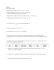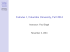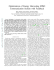# Document 6576713

## Transcription

Document 6576713
```2014‐15APCalculusABCh3MultipleChoiceProblems
1. lim → is
a) b)0c) d)1e)nonexistent
This does not appear to be the definition of a derivative, so let’s see if it is an indeterminate form. lim cos
2
2
→
1
1
0
0
0
0
0
Since this is an indeterminate form, we can use L’Hospital’s Rule. lim cos
2
2
→
sin
lim 2
→
1
2
2
0
2
0
2
1
2
AnswerC 2. 0. is increasing whenever 3 ∙
3 ∙
∙
2
∙
3
∙
always 3
3 ∙
∙2 1 critical values are need one factor positive, and one factor negative negative 3 0 only when 
3
0 (i.e., 3 ,and 
1
0 (i.e., 1 . 
Page | 1 These conditions occur simultaneously when: . AnswerD 1, 3
2014‐15APCalculusABCh3MultipleChoiceProblems
3. A differentiable function is increasing on any closed interval in which its first derivative is greater than zero on the corresponding open interval. Notice that the graph of is positive or zero on , . AnswerB Note: for purposes of determining an interval in which the function is increasing, the Increasing and Decreasing Interval Theorem implies that points where the first derivative is zero should be included in the interval. This is why the points at 2, 1, 3 are included in the interval in answer B. 4. Page | 2 A differentiable function is decreasing on any closed interval in which its first derivative is less than zero on the corresponding open interval. 0 on the open interval 2, 2 because it has exactly two zeros and we can see that they are at 2. So, we can conclude that is negative or zero on , . AnswerA
2014‐15APCalculusABCh3MultipleChoiceProblems
5. Let’s set the second derivative of the function equal to zero to find possible points of inflection. 3
5
15
20
60
60
60
1 Possible Inflection Points: 0, 1 Now we need to determine intervals around the possible inflection points and test the signs of the second derivative on those intervals. Actual inflection points exist where the second derivative changes sign. ∞,
,
,∞ : concave down A sign change in only at . concave down concave up 1, so there is an inflection point occurs only at the point where AnswerC
6. 1
2 Possible Inflection Points: 1, 0, 2 We need to determine intervals around the possible inflection points and test the signs of the second derivative on those intervals. Actual inflection points exist where the second derivative changes sign. ∞,
: ,
,
concave up concave down concave up ,∞ concave up A sign change in occurs at the points where 1, 0 , so there are inflection points only at and at . AnswerC Page | 3 2014‐15APCalculusABCh3MultipleChoiceProblems
7. ∙ cos
∙
cos
∙
sin
cos
∙2
2 ∙ cos
∙
cos
sin
∙2 Looking at a graph of the second derivative, we look for locations on the open interval 2, 2 where changes sign from positive to negative or from negative to poisitve. ,
This occurs five times on the interval . AnswerE 8. /
1
/
∙
1
/
∙
1
1
1
∙
/
∙
1
2
1
1
2
1
/
/
∙ 1
3
1
3
5
2√ 1
1
Looking at the graph of , we can see that changes sign from positive to negative somewhere near 0.5. The only answer in this neighborhood is AnswerB. Page | 4 2014‐15APCalculusABCh3MultipleChoiceProblems
9. A point of inflection exists where the second derivative of a function changes sign. Looking at the above graph, we can see that 0and changes sign at and . AnswerA 10. changes sign from positive to Using the First Derivative Test, we want ‐values where negative (a relative maximum) or from negative to positive (a relative minimum). 4 ∙
Set 0 to determine the critical numbers Next, look at a chart of the sign changes of ∞,
,
, remembering that ,∞ 2 and is negative. has a relative minimum at 2 and a relative maximum at 2. AnswerB Page | 5 2. 2014‐15APCalculusABCh3MultipleChoiceProblems
11. sin
1 If we are allowed to use a calculator to graph the function, we can simply plot the graph and count how many times it crosses the ‐axis. From the graph on the right, we see that this is four times. AnswerD
If we are not allowed to use a calculator to graph the function, we can still do this problem by noting that the sine function is zero for integer multiples of π. So, we can set: 1
0
1
1
2 1
3 , etc. The first equation, 1 0, has no solution, but the rest do. We just need to be sure to stay within the stated domain of 2
4. Then, we get: 1, √2
√
1, √3
1, √4
1, √5
1, √6
1 …}. The following values of are between 2 and 4: √2
1
2.299 √3
1
2.903 √4
1
3.401 √5
1
3.835 There are four of them. These are the four intersections with the ‐axis shown in the graph. Page | 6 2014‐15APCalculusABCh3MultipleChoiceProblems
12. The derivative shown appears to be a quadratic function. So, its associated function would be a cubic function. This immediately rules out Answer A and Answer B, which are lines. Notice that 2. 2. changes from negative to positive, indicating a relative minimum, at also changes from positive to negative, indicating a relative maximum, at The only graph with these characteristics is: AnswerE Page | 7 2014‐15APCalculusABCh3MultipleChoiceProblems
13. A relative maximum exists where the first derivative of a function changes from positive to negative. This happens for only, at the location shown by the magenta arrow. It does not happen for or . AnswerA 14. We are given: 0.1. We also know that We are asked to calculate: 2
, and so . ∙2 ∙
∙ 0.1
.
2
2014‐15APCalculusABCh3MultipleChoiceProblems
15. We are given:
3 and 3 For a triangle, 1
2
1
∙
2
∙
1
∙
2
∙
∙
3
3
2
∙3
So, increasing ⇒ 0 ⇒ decreasing ⇒ 0 ⇒ 0 ⇒ 0 ⇒ AnswerD 16. 3
Given:
sin
5
,so
5 cos ∙
When 3Find:
5 sin 3, we get: sin
and so cos
(recall: sin
cos
Then, 4
5∙ ∙3
5
AnswerE Page | 9 1) 2014‐15APCalculusABCh3MultipleChoiceProblems
17. /
1
2
1
/
2√
We want: 2∙
1
2∙
2√
1 1
2√1
Solve to get: AnswerA 18. 6
2
5 6
0 AnswerC Page | 10 2014‐15APCalculusABCh3MultipleChoiceProblems
19. Velocity increases when: So velocity increases when: 0. Note that 0, i.e., when
is concave upward. The curve is clearly concave upward on the left side of the graph. In addition, there is a point of inflection at 2. Therefore, the curve must be concave upward on the interval 0, 2 . AnswerA Page | 11 2014‐15APCalculusABCh3MultipleChoiceProblems
20. 3
3
6
6
12
4 6
12 0 ⇒ 1 is a critical number. In order to find the maximum acceleration on the closed interval 0, 3 , we must test the endpoints and the critical number. 0
3 0
6 0
12
12 1
3 1
6 1
12
9 which is the absolute minimum 3
3 3
6 3
12
which is the absolute maximum AnswerD 21. 7
1.01
1.01
∙ ln 1.01 ∙
2 ∙ ln 1.01 ∙ 1.01
1.01
∙ ln 1.01 ∙
2 3 ∙ ln 1.01 ∙ 1.01
.
AnswerB Page | 12 2 2014‐15APCalculusABCh3MultipleChoiceProblems
22. “Twice differentiable” means you can take second derivatives everywhere. The tangent line approximation is based on the following: ∆
∆
∙
So, 2
1
∆
1.9 and Solution: Let ∆
2 ∙
2 ∙
2 4 ∙ 1.9
2
2
∙
4
2
∙8
16 ∙
We want: 16 ∙
3 Subtract 3 from both sides of this equation so we can get an equation that we can solve using the calculator. 16 ∙
3
0 Graph this and solve for the value of on your calculator to get: .
. AnswerA Page | 13 2014‐15APCalculusABCh3MultipleChoiceProblems
24. 4
The line 2
graph of 3/4. So, we want the point on the 3 can be rewritten as that has a slope of . 1
2
We want , so we get rather quickly that . Now, let’s get the ‐coordinate: 1
2
1 1
2 2
1
8
Finally, the point we want is: ,
. AnswerB 25. At first blush, we might want to use L’Hospital’s Rule on this one. However, before we can do that, we need to see if the limit is indeterminate. lim
→
ln
1
0
This limit is NOT indeterminate because the numerator is not zero. The function increases without limit as → 1 from the right and decreases without limit as → 1 from the left. In cases like this we say the limit does not exist. AnswerE Page | 14 ```

### AP Calculus AB – Worksheet 83 The Second Derivative and The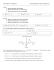### RQ 7 - Solutions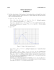### Math 120 Sample questions for Test 2, with answers### Worksheet #15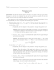### Week 6 notes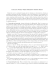### Don’t panic!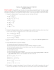### AP Calculus 5.4 Worksheet Day 1 All work must be shown in this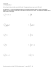### Calculus AB AP Sample Multiple-Choice Questions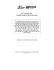### Calculus D Notes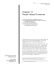### MATH 121, Calculus I — Sample Exam I (Fall 2013) Name: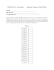### 1.6 Exam advice and sample questions for Chapter 1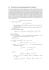### Calculus D Notes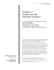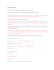### Math 120 Sample questions for Chapters 4&amp;5, with answers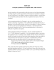### Math 220 Calculus I Exam 2 Fall 13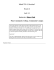### Name: AP